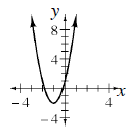### Home > CCA2 > Chapter 7 > Lesson 7.1.3 > Problem7-41

7-41.

Write the quadratic function in problem 7-40 in graphing form and sketch its graph.

Factor out the 3 and separate it from the constant.

$y = 3x^{2} + 6x + 1\\y = 3\left(x^{2} + 2x\right) + 1$

Complete the square.
Add the value that completes the square inside the parentheses
and subtract it outside.
(Why is the value subtracted 3 not 1?)

$y = 3\left(x^{2} + 2x + 1\right) + 1 − 3$

$y = 3\left(x + 1\right)^{2} − 2$